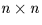Next: SLA_DMOON - Approx Moon Pos/Vel
Up: SUBPROGRAM SPECIFICATIONS
Previous: SLA_DM2AV - Rotation Matrix to Axial Vector

## SLA_DMAT - Solve Simultaneous Equations

ACTION:
Matrix inversion and solution of simultaneous equations (double precision).

CALL:
CALL sla_DMAT (N, A, Y, D, JF, IW)

GIVEN:

 I N number of unknowns A D(N,N) matrix Y D(N) vector

RETURNED:

 D(N,N) A matrix inverse Y D(N) solution D D determinant JF I singularity flag: 0=OK IW I(N) workspace

NOTES:
1.
For the set of n simultaneous linear equations in n unknowns:
Ay = x
where:
• A is a non-singularmatrix,
• y is the vector of n unknowns, and
• x is the known vector,
sla_DMAT computes:
• the inverse of matrix A,
• the determinant of matrix A, and
• the vector of n unknowns y.
Argument N is the order n, A (given) is the matrix A, Y (given) is the vector x and Y (returned) is the vector y. The argument A (returned) is the inverse matrix A-1, and D is det(A).
2.
JF is the singularity flag. If the matrix is non-singular, JF=0 is returned. If the matrix is singular, JF=-1 and D=0D0 are returned. In the latter case, the contents of array A on return are undefined.
3.
The algorithm is Gaussian elimination with partial pivoting. This method is very fast; some much slower algorithms can give better accuracy, but only by a small factor.
4.
This routine replaces the obsolete sla_DMATRX.Next: SLA_DMOON - Approx Moon Pos/Vel
Up: SUBPROGRAM SPECIFICATIONS
Previous: SLA_DM2AV - Rotation Matrix to Axial Vector

SLALIB --- Positional Astronomy Library